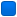###Autor Thema: Mein PID-Regler  (Gelesen 11912 mal)

0 Mitglieder und 1 Gast betrachten dieses Thema.

#### swmggs

• Gast##### Mein PID-Regler
« am: 26. Dezember 2006, 18:06:37 »
Hier mein PID-Regler, ohne Selbstoptimierung, die mache ich vorher.

mfg swmggs

####hugo

• Global Moderator
•• Beiträge: 2 143##### Re: Mein PID-Regler
« Antwort #1 am: 27. Dezember 2006, 00:02:42 »
hier der pid regler aus der oscat lib

FUNCTION_BLOCK FT_PID
VAR_INPUT
actual, set_point, offset, manual_in : REAL;
Manual : BOOL;
rst : BOOL := FALSE;
END_VAR
VAR_INPUT
KP, TN, TV, limit_L, limit_H : REAL;
END_VAR
VAR_OUTPUT
Y : REAL;
LIM, overflow : BOOL;
END_VAR
VAR
integ : FT_INT;
deriv : FT_deriv;
diff: REAL;
END_VAR

(*
version 1.1   25 dec 2006
programmer    hugo
tested by      tobias

FT_PID is a pid controller with manual functionality.
The PID controller works according to the fomula Y = KP * ( e + 1/TN * INTEG(e) + TV *DERIV(e)) + offset, while e = set_point - actual.
a rst will reset all internal data, while a switch to manual will cause the controller to follow the function Y = manual_in + offset.
limit_h and Limit_l set the possible output range of Y.
the output flags lim will signal that the output limits are active and overflow will signal that the integrator has reached the max or min limit.

since rev 1.1 the "trapezregel is used for more accuracy.
*)

IF rst OR KP = 0 THEN
y := offset;
integ(rst := 1);
integ.rst := 0;
ELSIF manual THEN
Y := manual_in + offset;
integ(rst := 1);
integ.rst := 0;
ELSE
(* calcualtion of the actual PID values *)
diff := set_point - actual;

(* integrator is only called if TN > 0 othewrwise it is disabled *)
IF TN > 0 THEN integ(in := diff, K := 1/TN, out_min := (limit_L - offset) / KP, out_max := (limit_H - offset) / KP); END_IF;
deriv(in := diff, K := TV);

(* check if integrator has reached the allowed limits and set overflow if necessary *)
IF integ.Out >= (limit_H - offset) / KP THEN
Y := limit_H;
overflow := TRUE;
ELSIF integ.Out <= (limit_L - offset) / KP THEN
Y := limit_L;
overflow := TRUE;
ELSE
Y := KP * (integ.Out + deriv.out + diff) + offset;
overflow := FALSE;
END_IF;

(* this portion limits the output to limit_H and limit_L and set the lim flag if limits are exceeded *)
IF Y > limit_H THEN
Y := limit_H;
Lim := TRUE;
ELSIF Y < limit_L THEN
Y := limit_L;
lim := TRUE;
ELSE
lim := FALSE;
END_IF;
END_IF;

#### swmggs

• Gast##### Re: Mein PID-Regler
« Antwort #2 am: 27. Dezember 2006, 09:26:29 »
Hallo Hugo,

hier ebenso, kennst ja mein Problem mit der englischen Sprache.

Für eine kurze deutsche Beschreibung wäre ich dankbar.

mfg. swmggs